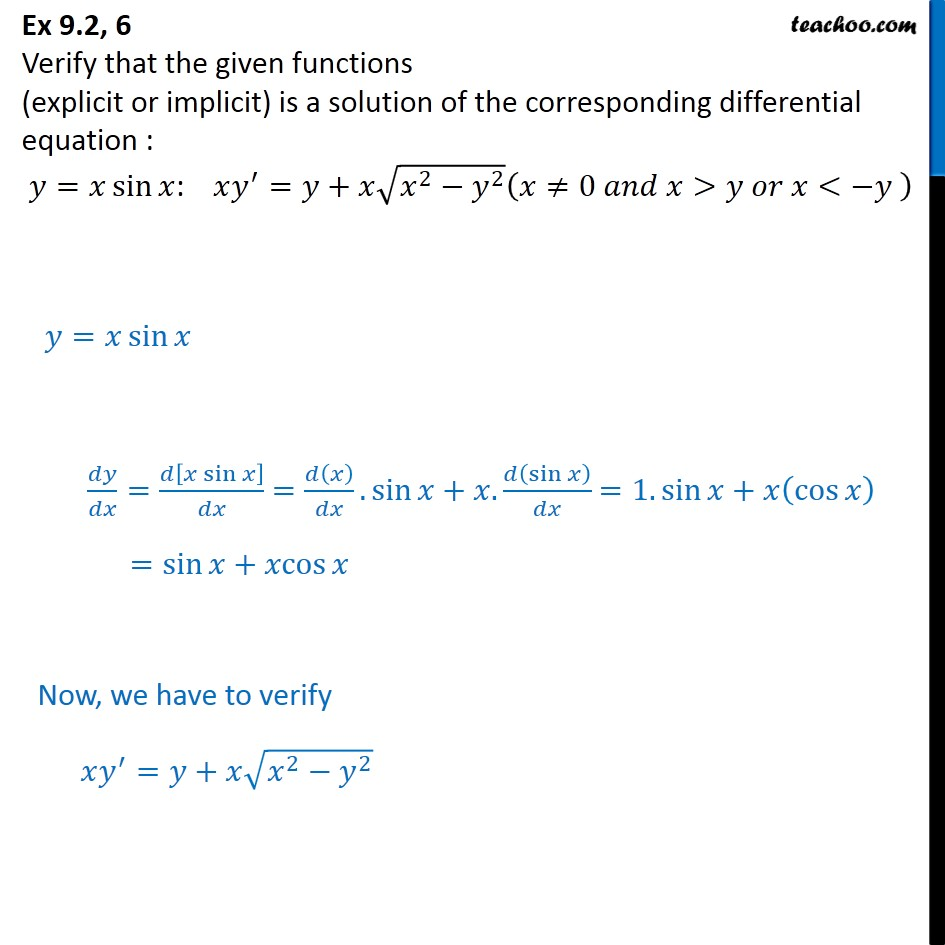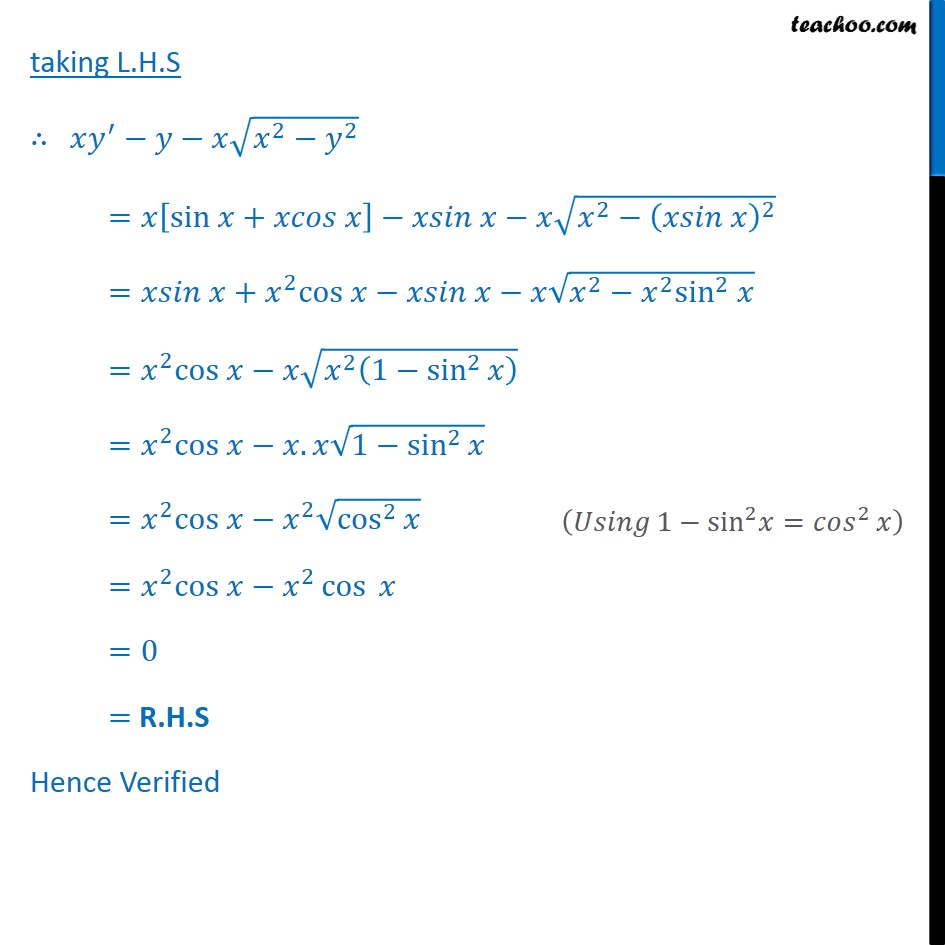1. Chapter 9 Class 12 Differential Equations
2. Serial order wise
3. Ex 9.2

Transcript

Ex 9.2, 6 Verify that the given functions (explicit or implicit) is a solution of the corresponding differential equation : 𝑦=𝑥 sin﷮𝑥﷯: 𝑥 𝑦﷮′﷯=𝑦+𝑥 ﷮ 𝑥﷮2﷯− 𝑦﷮2﷯﷯ 𝑥≠0 𝑎𝑛𝑑 𝑥>𝑦 𝑜𝑟 𝑥<−𝑦 ﷯ 𝑦=𝑥 sin﷮𝑥﷯ 𝑑𝑦﷮𝑑𝑥﷯= 𝑑 𝑥 sin⁡𝑥﷯﷮𝑑𝑥﷯ = 𝑑 𝑥﷯﷮𝑑𝑥﷯.sin⁡𝑥+𝑥. 𝑑 sin﷮𝑥﷯﷯﷮𝑑𝑥﷯ =1.sin⁡𝑥+𝑥 cos﷮𝑥﷯﷯ =sin⁡𝑥+𝑥cos⁡𝑥 Now, we have to verify 𝑥 𝑦﷮′﷯=𝑦+𝑥 ﷮ 𝑥﷮2﷯− 𝑦﷮2﷯﷯ taking L.H.S ∴ 𝑥 𝑦﷮′﷯−𝑦−𝑥 ﷮ 𝑥﷮2﷯− 𝑦﷮2﷯﷯ =𝑥 sin 𝑥+𝑥𝑐𝑜𝑠 𝑥﷯−𝑥𝑠𝑖𝑛 𝑥−𝑥 ﷮ 𝑥﷮2﷯− 𝑥𝑠𝑖𝑛 𝑥﷯﷮2﷯﷯ =𝑥𝑠𝑖𝑛 𝑥+ 𝑥﷮2﷯cos 𝑥−𝑥𝑠𝑖𝑛 𝑥−𝑥 ﷮ 𝑥﷮2﷯− 𝑥﷮2﷯ sin﷮2﷯ 𝑥﷯ = 𝑥﷮2﷯cos 𝑥−𝑥 ﷮ 𝑥﷮2﷯ 1− sin﷮2﷯﷮𝑥﷯﷯﷯ = 𝑥﷮2﷯cos 𝑥−𝑥.𝑥 ﷮1− sin﷮2﷯﷮𝑥﷯﷯ = 𝑥﷮2﷯cos 𝑥− 𝑥﷮2﷯ ﷮ cos﷮2﷯﷮𝑥﷯﷯ = 𝑥﷮2﷯cos 𝑥− 𝑥﷮2﷯ cos﷮ 𝑥﷯ =0 = R.H.S Hence Verified

Ex 9.2